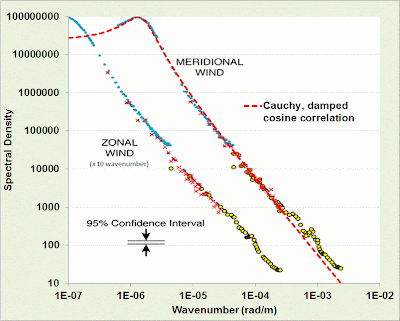## Context/Earth

[[ Check out my Wordpress blog Context/Earth for environmental and energy topics tied together in a semantic web framework ]]

## Monday, March 18, 2013

### Spatial and Temporal Correlations of Wind

In terms of climate statistics, we always have more data than we know what to do with. The challenge is in reducing the data into meaningful bits of information which can be characterized and modeled succinctly.

So this is a case of where enough data exists that we can get an understanding of the spatial and temporal correlations of prevailing winds.

One source of data comes from measurements of winds at altitude, taken by jet airliners as part of their regular flight routes and then post-processed and analyzed. This gives an indication of spatial correlation over a large dynamic range, stretching a little over three orders of magnitude.  Once collected, the post-processing involved applying an autocorrelation to the data over all spatial scales.  The power spectral density (PSD) is the Fourier transform of the autocorrelation, and that is shown in Figure 1 below, along with a suggested model achieving a good fit to the actual meridonal wind PSD.Figure 1 : Zonal and Meridional wind speed PSD. The dashed line model fit works well apart from deviations near the noisy shorter wavelengths.
The chosen model is simply the PSD of an exponentially damped cosine autocorrelation (see  for examples in meteorology).

$$C(x) = e^{-\alpha |x|} \cdot cos(\beta x)$$

The Fourier transform of this autocorrelation gives a PSD that is best described as a shifted Cauchy or Lorentzian profile.  In Figure 1, the shape is obvious as it shows a peak and then a concave-upward inflection following the peak.

$$I(S_x) = \frac{\alpha}{(S_x-\beta)^2 + \alpha^2}$$

This specific profile is generated by a semi-Markovian process.  The "semi" part captures the periodic portion while the Markov contributes the memory for close-proximity coupling. Specifically, the Markov term is the alpha-factor damping which gives the overall 1/S^2 roll-off, while the beta-spatial shift indicates a semi-Markov ordering feature indicating some underlying longer-range spatial periodicity.

$$\beta = 2 \pi/L = \text{1.3e-6 rad/m}$$
$$\alpha = \text{7.63e-7 rad/m}$$

This gives a weak periodicity of L=4833 km.   For a prevailing wind-stream of 30 MPH, this spans approximately 4 days of flow between a peak and a lull in wind speed.

The spatial correlation intuitively leads to the concept of a temporal correlation in wind speed, and the question of whether this characteristic can be measured and contrasted to the spatial correlation.

For temporal correlation, I used the same data from the BPA which I had previously applied to a probability density function (PDF) analysisFigure 2 : Temporal autocorrelation of wind speed
The temporal autocorrelation of the Roosevelt Island site shows some periodic fine structure. Using the Eureqa equation fitting software, I get the following empirical autocorrelation match.

$$c(t)= 0.02934 \cdot sin(0.5012 - 1.626 t) + 0.01668 \cdot cos(0.8645 + 6.203 t) + exp(-1.405 t) - 0.02134 \cdot sin(1.088 - 0.599 t)$$

The first and second terms are periodicities of 3.86 and 1 days, the latter easily explained by daily (diurnal) variations.  There is also a strong damping factor with a half-life less than a day. The last term is a 10 day period which generates a longer term modulation (and also has more uncertainty in its weighting).

The 3.86 day periodicity is likely due to a principle oscillation pattern in unstable meandering baroclinic Rossby waves as detected through a separate statistical analysis 

This demonstrates how the spatial correlation relates to the temporal correlation. As the tropospheric wave patterns propagate and disperse, they influence the local wind patterns.  These are quite subtle effects yet they can be detected and perhaps can be put to good use in battling the intermittency in wind power.

#### References

 G. Nastrom and K. S. Gage, “A climatology of atmospheric wavenumber spectra of wind and temperature observed by commercial aircraft,” Journal of the atmospheric sciences, vol. 42, no. 9, pp. 950–960, 1985.

 H. J. Thiebaux, “Experiments with correlation representations for objective analysis,” Monthly Weather Review, vol. 103, p. 617, 1975.

 H. Von Storch and F. W. Zwiers, Statistical analysis in climate research. Cambridge University Press, 2002.